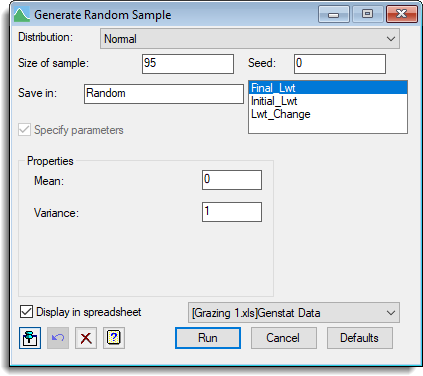1. Home
2. Generating a Random Sample from a Distribution

# Generating a Random Sample from a Distribution

You can generate a pseudo-random sample from a selected distribution and store the results in a new column in your spreadsheet. The distribution can be specified in terms of its mean and variance, or in some cases by the parameters of the distribution.2. Select a Distribution to draw the sample from.
3. Set the Seed value used to generate the first random number.
You can reuse this value later to ensure that the same random numbers are produced. If set to zero a new random number will be generated each time.
4. Enter a name for the sample variate in the Save In field.
5. If required, specify the parameters of the distribution.
These fields will change depending on which distribution you selected.
6. Set other options as required then click Run.

If you selected to display the column in a spreadsheet it will appear with a half-yellow background to indicate that this is a calculated column.Distribution Specifies the distribution from which the sample is to be drawn. Beta Chi-square Exponential F Gamma Log-normal Normal t Uniform Weibull Binomial Hypergeometric Poisson Size of sample Sets the size of sample to be generated (the number of rows). Seed Sets the seed for the random number generator. A value of 0 will use a random seed generated from the system clock if this is the first use of the generator; otherwise it will continue the sequence previously used. If the seed is taken from the system clock a message will be produced in the output indicating its value, allowing it to be re-used. Any particular non-zero integer seed will always produce the same underlying sequence of pseudo-random numbers before transformation to the required distribution, so the seed should normally be set to 0 except when you wish to exactly repeat the random sample. Save in Specifies the name of a variate to save the sample in. Specify parameters Lets you specify the selected distribution in terms of its parameters. Properties Lets you define the distribution according to various moments or parameters depending on the setting of Specify parameters. Click the link for a Distribution to see a description of how it may be defined. Display in spreadsheet Lets you display the results in a spreadsheet. You can select the sheet from the list of current open spreadsheets or request a new spreadsheet be created. Note: the number of rows of the spreadsheet must match the length of the results formed by the calculation, otherwise a new sheet will be used.
Updated on June 20, 2019